# Electronics and Communication Engineering - Exam Questions Papers

31.

If the system T.F. is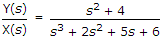The differential equation representing the system is

 A.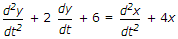B.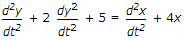C.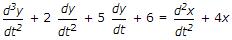D. none of these

Explanation: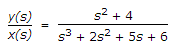(s3 + 2s2 + 5s + 6)y(s) = (s2 + 4) x (s)

s3y(s) + 2s2y(s) + 5sy(s) + 6y(s) = s2 x (s) + 4 x (s)

Replacing s by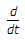and y(s) by y(t) and x(s) by x(t) we get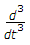y(t) + 2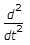y(t) + 5y(t) + 6y(t)

=x(t) + 4x(t)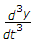+ 2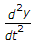+ 5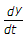+ 6 =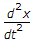+ 4x

This is required differential equation.

32.

In the circuit below, delay of the EXOR and AND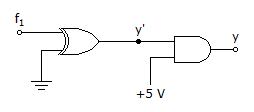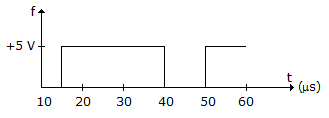Logic gates are 20 μs and 10 μs respectively, then the wave y' and y (with initial condition y = 1) will be :

 A.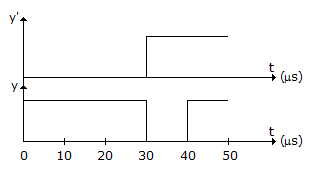B.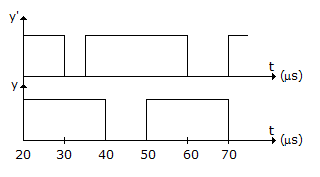C.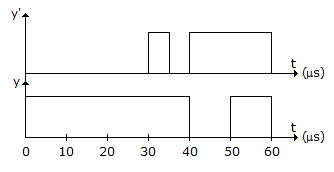D.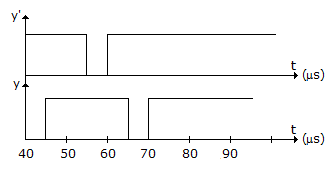Explanation:

Since y is at logic '1' initially, y' must be at logic '1'.

Also, one input of the EXOR gate is tied to zero therefore 'f' will be directly transferred to the output.

The logic gates will introduce gate delays which will lead to the answer .

33.

The antenna current of an AM transmitter is 8 A, when only the carrier is sent, but it increases to 8.93 A when the carrier is modulated by a single sine wave. Determine the antenna current when the percent of modulation changes to 0.8

 A. 9.19 A B. 9.91 A C. 10.56 A D. 8.61 A

Explanation:

Ic = 8 A

m = 0.8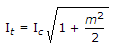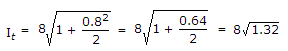= 8 x 1.149 = 9.19 A.

34.

A noiseless 3 KHz channel can transmit binary at the rate __________ .

 A. 12000 bps B. 10000 bps C. 6000 bps D. 3000 bps

Explanation:

Maximum data rate for noiseless channel is given by Nyquist Theorem

= 2H log2 V bits/sec

V - discrete levels (Here Binary i.e. 2)

H - Bandwidth

Maximum data rate = 2H log2 V

= 2(3 KHz) log2 2 = 2 x 3 x 103 x 1

= 6 x 103 = 6000 bps.

35.

Find Vx Vy Vz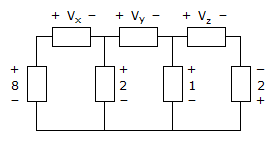A. Vx = -6 Vy = 3 Vz = -3 B. Vx = -6 Vy = -3 Vz = 1 C. Vx = 6 Vy = 3 Vz = 3 D. Vx = 6 Vy = 1 Vz = 3

Explanation:

Apply Mesh analysis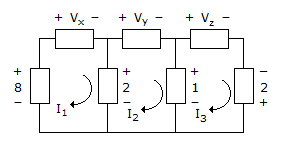Apply KVL to mesh 2

-2 + Vy + 1 = 0

Vy = 1

Apply KVL to mesh 1

-8 + Vx + 2 = 0

Vx = 6 V

Apply KVL to mesh 3

-1 + Vz -2 = 0

Vz = 3 V.